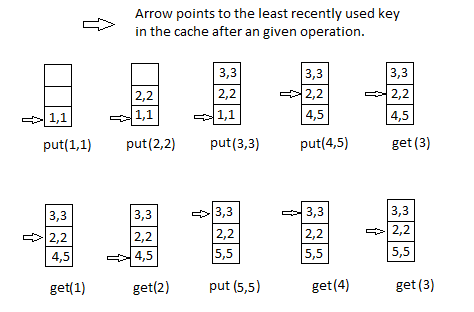New update is available. Click here to update.

# LRU Cache Implementation

Last Updated: 29 Aug, 2020
Difficulty: Moderate

## PROBLEM STATEMENT

#### Design and implement a data structure for Least Recently Used (LRU) cache to support the following operations:

``````1. get(key) - Return the value of the key if the key exists in the cache, otherwise return -1.

2. put(key, value), Insert the value in the cache if the key is not already present or update the value of the given key if the key is already present. When the cache reaches its capacity, it should invalidate the least recently used item before inserting the new item.
``````
##### You will be given ‘Q’ queries. Each query will belong to one of these two types:
``````Type 0: for get(key) operation.
Type 1: for put(key, value) operation.
``````
##### Note :
``````1. The cache is initialized with a capacity (the maximum number of unique keys it can hold at a time).

2. Access to an item or key is defined as a get or a put operation on the key. The least recently used key is the one with the oldest access time.
``````
##### Input Format :
``````The first line of input contains two space-separated integers 'C' and 'Q', denoting the capacity of the cache and the number of operations to be performed respectively.

The next Q lines contain operations, one per line. Each operation starts with an integer which represents the type of operation.

If it is 0, then it is of the first type and is followed by one integer key.

If it is 1, it is of the second type and is followed by two space-separated integers key and value(in this order).
``````
##### Output Format :
``````For each operation of type 0, print an integer on a single line, denoting the value of the key if the key exists, otherwise -1.
``````
##### Note :
``````You don't need to print anything, it has already been taken care of. Just implement the given function.
``````
##### Constraints :
``````1 <= C <= 10^4
1 <= Q <= 10^5
1 <= key, value <= 10^9

Time Limit: 1 sec
``````
##### Sample Input 1 :
``````3 11
1 1 1
1 2 2
1 3 3
1 4 5
0 3
0 1
0 4
1 2 3
0 1
0 3
0 2
``````
##### Sample Output 1 :
``````3
-1
5
-1
3
3
``````
##### Explanation to Sample Input 1 :``````Initializing a cache of capacity 3, LRUCache cache = new LRUCache(3);
Then each operation is performed as shown in the above figure.
cache.put(1,1)
cache.put(2,2)
cache.put(3,3)
cache.put(4,5)
cache.get(3)      // returns 3
cache.get(1)      // returns -1
cache.get(2)      // returns 2
cache.put(5,5)
cache.get(4)      // returns -1
cache.get(3)      // returns 3
``````
##### Sample Input 2 :
``````2 6
1 1 1
1 2 2
0 2
1 3 3
0 3
0 1
``````
##### Sample Output 2 :
``````2
3
-1
``````